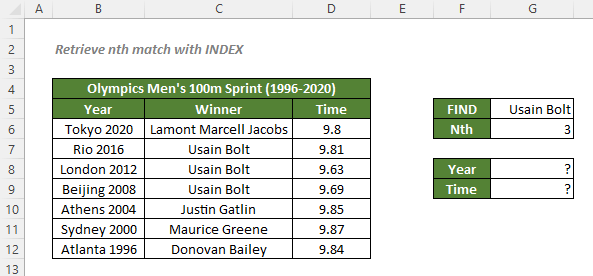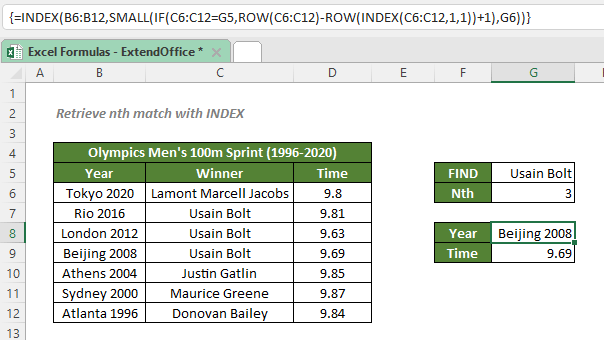## Retrieve nth match with INDEX

To find the nth match of a value from a range and retrieve its corresponding data, you can use a formula based on the INDEX, ROW, SMALL, and IF functions.#### How to retrieve information associated with the nth match of a value with INDEX?

To get the information about the 3rd match of Usain Bolt in the table as shown above, you can use the ROW and IF functions together to get an array that consists of the row numbers of all the Usain Bolt values. Then use the SAMLL function to retrieve the row number of the nth matched value you specified. Finally, feed the result to the INDEX function to get the corresponding information at the same row.

#### Generic syntax

=INDEX(return_range,SMALL(IF(lookup_array=lookup_value,ROW(lookup_array-ROW(INDEX(lookup_array,1,1))+1),n))

√ Note: This is an array formula that requires you to enter with Ctrl + Shift + Enter.

• return_range: The range to return the information corresponding to the nth match from. Here refers to the year or time range.
• lookup_array: The range to look for the row position of the nth match from. Here refers to the winner range.
• lookup_value: The value you specified to lookup its nth match. Here refers to Usain Bolt.
• n: Indicate the nth match. To find the 1st match of a value, set n as 1; to find the 2nd match, set n as 2.

To get the information about the 3rd match of Usain Bolt, please copy or enter the formulas below in the cells G8 and G9, and press Ctrl + Shift + Enter to get the results:

Year (Cell G8)
=INDEX(B6:B12,SMALL(IF(C6:C12=G5,ROW(C6:C12-ROW(INDEX(C6:C12,1,1))+1),G6))
Time (Cell G9)
=INDEX(D6:D12,SMALL(IF(C6:C12=G5,ROW(C6:C12-ROW(INDEX(C6:C12,1,1))+1),G6))#### Explanation of the formula

Here we use the formula below as an example:

=INDEX(B6:B12,SMALL(IF(C6:C12=G5,ROW(C6:C12)-ROW(INDEX(C6:C12,1,1))+1),G6))

• ROW(C6:C12): The ROW function returns the row numbers of each cells in the range C6:C12 in an array like this: {6;7;8;9;10;11;12}.
• ROW(INDEX(C6:C12,1,1)): The INDEX and ROW functions return the first row number of the lookup range C6:C12 in this Excel worksheet, which is 6.
• IF(C6:C12=G5,ROW(C6:C12)-ROW(INDEX(C6:C12,1,1))+1) = IF(C6:C12=G5,{6;7;8;9;10;11;12}-6+1): The IF function checks if each value in the range C6:C12 matches the value in the cell G5, Usain Bolt. If yes, the function will return the result of “its corresponding row number-6+1”; Otherwise return a FALSE. So the results would be in an array like this: {FALSE;2;3;4;FALSE;FALSE;FALSE}.
• SMALL(IF(C6:C12=G5,ROW(C6:C12)-ROW(INDEX(C6:C12,1,1))+1),G6) = SMALL({FALSE;2;3;4;FALSE;FALSE;FALSE},G6): The snippet returns the 3rd (value in cell G6) smallest value from the array, which is 4.
• INDEX(B6:B12,SMALL(IF(C6:C12=G5,ROW(C6:C12)-ROW(INDEX(C6:C12,1,1))+1),G6)) = INDEX(B6:B12,4) The INDEX function returns the 4th value in the year range B6:B12, which is Beijing 2008.

#### Related functions

Excel INDEX function

The Excel INDEX function returns the displayed value based on a given position from a range or an array.

Excel IF function

The IF function is one of simplest and most useful functions in Excel workbook. It performs a simple logical test which depending on the comparison result, and it returns one value if a result is TRUE, or another value if result is FALSE.

Excel ROW function

The Excel ROW function returns the row number of a reference.

#### Related Formulas

Retrieve nth match with VLOOKUP

Suppose you have a list of values and their corresponding information, to retrieve the related information of the nth match of a value, you can use a formula based on the VLOOKUP function.

Retrieve information associated with lowest n values

To retrieve data that are corresponding to the smallest, 2nd smallest, or nth smallest value in a list, table or row in Excel, you can use an INDEX and MATCH formula together with the SMALL function.

Get information corresponding to maximum value

To retrieve data that are corresponding to the maximum value in a list, table or row in Excel, you can use an INDEX and MATCH formula together with the MAX function.

Get information corresponding to minimum value

To retrieve data that are corresponding to the minimum value in a table, you can use an INDEX and MATCH formula together with the MIN function.

### The Best Office Productivity Tools

#### Kutools for Excel - Helps You To Stand Out From Crowd

 Popular Features: Find, Highlight or Identify Duplicates  |  Delete Blank Rows  |  Combine Columns or Cells without Losing Data  |  Round without Formula ... Super VLookup: Multiple Criteria  |  Multiple Value  |  Across Multi-Sheets  |  Fuzzy Lookup... Adv. Drop-down List: Easy Drop Down List  |  Dependent Drop Down List  |  Multi-select Drop Down List... Column Manager: Add a Specific Number of Columns  |  Move Columns  |  Toggle Visibility Status of Hidden Columns  |  Compare Columns to Select Same & Different Cells ... Featured Features: Grid Focus  |  Design View  |  Big Formula Bar  |  Workbook & Sheet Manager | Resource Library (Auto Text)  |  Date Picker  |  Combine Worksheets  |  Encrypt/Decrypt Cells  |  Send Emails by List  |  Super Filter  |  Special Filter (filter bold/italic/strikethrough...) ... Top 15 Toolsets:  12 Text Tools (Add Text, Remove Characters ...)  |  50+ Chart Types (Gantt Chart ...)  |  40+ Practical Formulas (Calculate age based on birthday ...)  |  19 Insertion Tools (Insert QR Code, Insert Picture from Path ...)  |  12 Conversion Tools (Numbers to Words, Currency Conversion ...)  |  7 Merge & Split Tools (Advanced Combine Rows, Split Excel Cells ...)  |  ... and more

Kutools for Excel Boasts Over 300 Features, Ensuring That What You Need is Just A Click Away...#### Office Tab - Enable Tabbed Reading and Editing in Microsoft Office (include Excel)

• One second to switch between dozens of open documents!
• Reduce hundreds of mouse clicks for you every day, say goodbye to mouse hand.
• Increases your productivity by 50% when viewing and editing multiple documents.
• Brings Efficient Tabs to Office (include Excel), Just Like Chrome, Edge and Firefox.